# Confidence vs. Credibility Intervals

Tomorrow, for the final lecture of the Mathematical Statistics course, I will try to illustrate – using Monte Carlo simulations – the difference between classical statistics, and the Bayesien approach.

The (simple) way I see it is the following,

• for frequentists, a probability is a measure of the the frequency of repeated events, so the interpretation is that parameters are fixed (but unknown), and data are random
• for Bayesians, a probability is a measure of the degree of certainty about values, so the interpretation is that parameters are random and data are fixed

Or to quote Frequentism and Bayesianism: A Python-driven Primer,  a Bayesian statistician would say “given our observed data, there is a 95% probability that the true value of $\theta$ falls within the credible region” while a Frequentist statistician would say “there is a 95% probability that when I compute a confidence interval from data of this sort, the true value of $\theta$ will fall within it”.

To get more intuition about those quotes, consider a simple problem, with Bernoulli trials, with insurance claims. We want to derive some confidence interval for the probability to claim a loss. There were = 1047 policies. And 159 claims.

Consider the standard (frequentist) confidence interval. What does that mean that $$\overline{x}\pm\sqrt{\frac{\overline{x}(1-\overline{x})}{n}}$$is the (asymptotic) 95% confidence interval? The way I see it is very simple. Let us generate some samples, of size $n$, with the same probability as the empirical one, i.e. $\widehat{\theta}$ (which is the meaning of “from data of this sort”). For each sample, compute the confidence interval with the relationship above. It is a 95% confidence interval because in 95% of the scenarios, the empirical value lies in the confidence interval. From a computation point of view, it is the following idea,

> xbar <- 159
> n <- 1047
> ns <- 100
> M=matrix(rbinom(n*ns,size=1,prob=xbar/n),nrow=n)

I generate 100 samples of size . For each sample, I compute the mean, and the confidence interval, from the previous relationship

> fIC=function(x) mean(x)+c(-1,1)*1.96*sqrt(mean(x)*(1-mean(x)))/sqrt(n)
> IC=t(apply(M,2,fIC))
> MN=apply(M,2,mean)

Then we plot all those confidence intervals. In red when they do not contain the empirical mean

> k=(xbar/n<IC[,1])|(xbar/n>IC[,2])
> plot(MN,1:ns,xlim=range(IC),axes=FALSE,
+ xlab="",ylab="",pch=19,cex=.7,
+ col=c("blue","red")[1+k])
> axis(1)
> segments(IC[,1],1:ns,IC[,2],1:
+ ns,col=c("blue","red")[1+k])
> abline(v=xbar/n)Now, what about the Bayesian credible interval ? Assume that the prior distribution for the probability to claim a loss has a distribution. We’ve seen in the course that, since the Beta distribution is the conjugate of the Bernoulli one, the posterior distribution will also be Beta. More precisely

Based on that property, the confidence interval is based on quantiles of that (posterior) distribution

> u=seq(.1,.2,length=501)
> v=dbeta(u,1+xbar,1+n-xbar)
> plot(u,v,axes=FALSE,type="l")
> I=u<qbeta(.025,1+xbar,1+n-xbar)
> polygon(c(u[I],rev(u[I])),c(v[I],
+ rep(0,sum(I))),col="red",density=30,border=NA)
> I=u>qbeta(.975,1+xbar,1+n-xbar)
> polygon(c(u[I],rev(u[I])),c(v[I],
+ rep(0,sum(I))),col="red",density=30,border=NA)
> axis(1)What does that mean, here, that we have a 95% credible interval. Well, this time, we do not draw using the empirical mean, but some possible probability, based on that posterior distribution (given the observations)

> pk <- rbeta(ns,1+xbar,1+n-xbar)

In green, below, we can visualize the histogram of those values

> hist(pk,prob=TRUE,col="light green",
+ border="white",axes=FALSE,
+ main="",xlab="",ylab="",lwd=3,xlim=c(.12,.18))

And here again, let us generate samples, and compute the empirical probabilities,

> M=matrix(rbinom(n*ns,size=1,prob=rep(pk,
+ each=n)),nrow=n)
> MN=apply(M,2,mean)

Here, there is 95% chance that those empirical means lie in the credible interval, defined using quantiles of the posterior distribution. We can actually visualize all those means : in black the mean used to generate the sample, and then, in blue or red, the averages obtained on those simulated samples,

> abline(v=qbeta(c(.025,.975),1+xbar,1+
+ n-xbar),col="red",lty=2)
> points(pk,seq(1,40,length=ns),pch=19,cex=.7)
> k=(MN<qbeta(.025,1+xbar,1+n-xbar))|
+ (MN>qbeta(.975,1+xbar,1+n-xbar))
> points(MN,seq(1,40,length=ns),
+ pch=19,cex=.7,col=c("blue","red")[1+k])
> segments(MN,seq(1,40,length=ns),
+ pk,seq(1,40,length=ns),col="grey")More details and exemple on Bayesian statistics, seen with the eyes of a (probably) not Bayesian statistician in my slides, from my talk in London, last Summer,

# Binomial regression model

Most of the time, when we introduce binomial models, such as the logistic or probit models, we discuss only Bernoulli variables, . This year (actually also the year before), I discuss extensions to multinomial regressionswhere  is a function on some simplex. The multinomial logistic model was mention here. The idea is to consider, for instance with three possible classes

the following model

and

# In three months, I’ll be in Vegas (trying to win against the house)In fact, I’m going there with my family and some friends, including two probabilists (I mean professionals, I am merely an amateur), with this incredible challenge: will I be able to convince  probabilists to go to play at the Casino?

Actually, I also want to study them carefully, to understand how we should play optimally. For example, I hope I can make them play the roulette. Roulette is simple. With a French (or European) roulette, it is probably the simplest: if I bet on black, I win if one of 18 black numbers is out, and I lose if one of the 18 red numbers – or zero (which is green) – is out. This gives a winning probability of 18/37 i.e. a 48.64% chance. But in Vegas, I think it’s mostly American Roulette that can be found in casinos, in which there is a zero and a double zero (both favorable to the bank). Here, the  probability of winning is 18/38, i.e. 47.36% chance. The two roulettes areNow, let us discuss a little bit about optimal strategy. For instance, suppose I go to Las Vegas with an initial wealth  (say $100). The goal is to find the strategy which maximizes the probability to leave Las Vegas with (here$200). Should I play big, or small ?

Assume that I can bet  (that will be, here, for convenience, a fraction of ). With probability , I will get , and with probability , I will get  (and lose my ). As mentioned above,  is (a little) smaller than 50%. The casino must win (actually, we will see that this assumption has a very strong impact on the strategy).

Suppose my goal is to double my initial sum, as mentioned in the introduction of this post. Maybe there is an optimum value for , to maximize the probability of doubling my bet. To make it simple, the game ends either because I did not, or because I did, manage to double my initial wealth… Assume further that  is fixed, and that I do not revise my bets. One can use monte carlo simulations, to get an intuitive idea…

> bet=function(s=1,t=2*s,x=s/4,p=.4736,nsim=100000){
+     vp=rep(0,nsim); #vw=s
+     for(i in 1:nsim){
+       w=s;
+       while((w>0)&(w<t)){
+          ux=sample(c(min(x,t-w),-x),size=1,prob=c(p,1-p))
+          w=w+ux
+       }
+       vp[i]=(w>=t)}
+     return(mean(vp))
+ }

If we plot this probability as a function of , we have the following

> BET=function(x) bet(x=x)
> vx=1/(1:20)
> px= Vectorize(BET)(vx)
> plot(vx,px,log="x")Let us see if we can do the maths, and actually compute those probabilities.

For example, if , I play everything I have, and I double with probability . That one was simple.  And indeed, on the graph above, the point on the right is probability   (the red horizontal line).

Assume now that I can bet , and I will play, at least, two rounds

• with probability  I will lose both rounds (and the game is over)
• with probability , I will win both rounds, and I double my bet (and the game is also over)
• with probability , I will lose once, and double once. Anyway, I will find myself again with my (initial) wealth . So the game will start again….

To make the story short the probability of doubling my earnings is

which is

Let’s try something more general: I have initial wealth , I can bet  and the goal is to reach  (or, more generally, say, ). Now, the probability to reach  from  betting (always)  is exactly the same as the probability to reach  from  betting only 1. Let  denote the probability to go from  to  betting 1 (let us use generic parameters). We can easily get the following equation

Thus, we can write

or equivalently

Now, observe that  (since I cannot have a gain without any money).

Let us write  using a domino technique :

i.e.

so this geometric sum can also be written

Finally, we can write

Here, there is still  that I have to explicit. The idea is to observe that , thus

So finally,

Nice isn’t it? But to be honest, there is nothing new here. This is actually an old theorem discovered by Christiaan Huygens in 1657, then extended by Jacob Bernoulli in 1680 and finally properly established by Abraham de Moivre in 1711. It is possible to plot this graph, as a function of ,

> bet2=function(s=1,t=2*s,x=s/4,p=.4736){
+     vp=(1-((1-p)/p)^(s/x))/(1-((1-p)/p)^(t/x))
+     return(vp)
+ }The graph is the same as the one with monte carlo simulation (hopefully). Observe, looking carefully at the function above, that the probability is decreasing with . Which makes sense… Further, the probability is decreasing with : the more hungry, the less chance of winning I have.

Now, the interesting part is what is plotted on the graphs above: the smaller  (the size of the bets at each round), the less chances to win: if I want to win, it is important not to play being little player ! I must bet everything I have ! Actually, the funny thing is that if the probability of winning was (slightly) larger than 1/2, on the contrary, I should bet as small as possibleSo far, there is nothing new. Everything mentioned in this post can be related to a fundamental result of Lester Dubins and Leonard Savage, in “How to Gamble if You Must : Inequalities for Stochastic Processes” (published in 1965), see also Sudderth (1972). Of course, I can try another strategy, a little less reasonable, I think, which is sometimes called Martingale of D’Alembert. I believe more in luck than coincidence, so, when I win, I drop my bet (do not tempt fate) but when I lose, I increase my bet (I must win someday). But let’s keep it for another post, someday…

Again, that’s a theory. I guess we should try, and see how it works. I’ll try to upload pictures on the blog during the road trip, so if by the beginning in August nothing has been posted on the blog, please send a rescue team to save me at the Bellagio…

# Will I ever be a bayesian statistician ? (part 1)

Last week, during the workshop on Statistical Methods for Meteorology and Climate Change (here), I discovered how powerful bayesian techniques could be, and that there were more and more bayesian statisticians. So, if I was to fully understand applied statisticians in conferences and workshops, I really have to understand basics of bayesian statistics. I have published some time ago some posts on bayesian statistics applied to actuarial problems (here or there), but so far, I always thought that bayesian was a synonym for magician. To be honest, I am a Muggle, and I have not been trained as a bayesian. But I can be an opportunist…

So I decided to publish some posts on bayesian techniques, in order to prove that it is actually not that difficult to implement.As far as I understand it, in bayesian statistics, the parameter is considered as a random variable (which is also the case, in classical mathematical statistics). But here, here assume that this parameter does have a parametric distribution….
Consider a classical statistical problem: assume we have a sample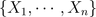i.i.d. with distribution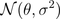. Here we note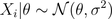since parameteris a random variable. The idea is to assume thathas a (so called a priori) distribution, e.g.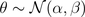So far it was simple. The idea is then to consider the posterior distribution of, given the observations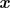. Thus, we need to compute the distribution of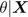which is here extremely simple (due to properties of the Gaussian distribution), i.e.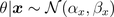where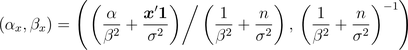And them, it becomes extremely natural to consider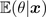as an estimator of given our sample data (and thus, we also have a confidence interval since we know the distribution ofgiven the observations).
In order to be sure that we understood, consider now a heads and tails problem, i.e.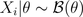. Note, first, that \theta has support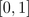. So we need a distribution on that support. Why not a beta distribution ? E.g.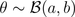Thus,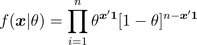and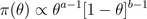From Bayes formula,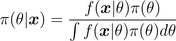and we get easily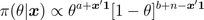which is the density of a Beta distribution, i.e.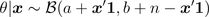prior=dbeta(u,a,b)
posterior=dbeta(u,a+y,n-y+b)

The estimator proposed is then the expected value of that conditional distribution,Note that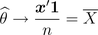Further, it is possible to derive confidence intervals using quantiles of the posterior distribution.
On the graphs below, we consider the following heads/tails sampleA first idea is to consider a uniform prior distribution.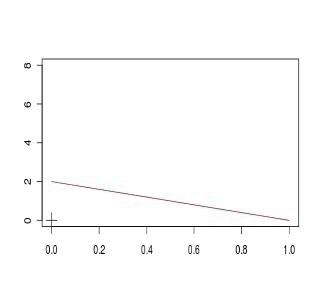A second idea is to consider an asymmetric beta distribution. First, with an asymmetry on the left,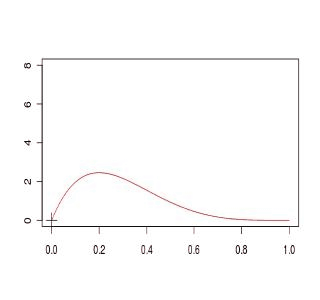or on the right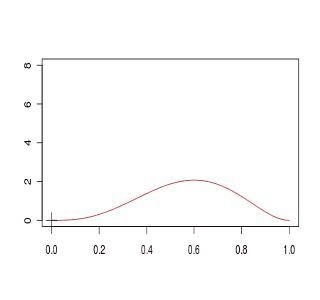Finally a third idea is simply to get back to the standard Gaussian approximation,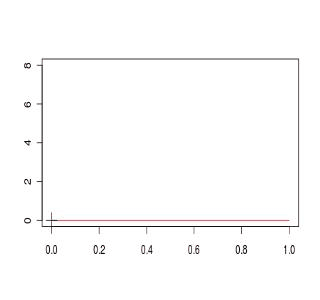If we compare the four models, we obtain (the plain black line is the Gaussian approximated distribution for the empirical mean), and red lines are obtained from prior beta distributionsThe code to generate those graphs is the following
a1=1; b1=1
D1[1,]=dbeta(u,a,b)
a2=4; b2=2
D2[1,]=dbeta(u,a,b)
a3=2; b3=4
D3[1,]=dbeta(u,a,b)
setseed(1)
S=sample(0:1,size=100,replace=TRUE)
COULEUR=rev(rainbow(120))
D1=D2=D3=D4=matrix(NA,101,length(u))
for(s in 1:100){
y=sum(S[1:s])
D1[s+1,]=dbeta(u,a1+y,s-y+b1)
D2[s+1,]=dbeta(u,a2+y,s-y+b2)
D3[s+1,]=dbeta(u,a3+y,s-y+b3)
D4[s+1,]=dnorm(u,y/s,sqrt(y/s*(1-y/s)/s))
plot(u,D1[1,],col="black",type="l",ylim=c(0,8),
xlab="",ylab="")
for(i in 1:s){lines(u,D1[1+i,],col=COULEUR[i])}
points(y/s,0,pch=3,cex=2)
plot(u,D2[1,],col="black",type="l",ylim=c(0,8),
xlab="",ylab="")
for(i in 1:s){lines(u,D2[1+i,],col=COULEUR[i])}
points(y/s,0,pch=3,cex=2)
plot(u,D3[1,],col="black",type="l",ylim=c(0,8),
xlab="",ylab="")
for(i in 1:s){lines(u,D3[1+i,],col=COULEUR[i])}
points(y/s,0,pch=3,cex=2)
plot(u,D4[1,],col="white",type="l",ylim=c(0,8),
xlab="",ylab="")
for(i in 1:s){lines(u,D4[1+i,],col=COULEUR[i])}
points(y/s,0,pch=3,cex=2)
plot(u,D4[s+1,],col="black",lwd=2,type="l",
ylim=c(0,8),xlab="",ylab="")
lines(u,D1[1+i,],col="blue")
lines(u,D2[1+i,],col="red")
lines(u,D3[1+i,],col="purple")
points(y/s,0,pch=3,cex=2)
}

Here, we can see that computations are simple if the prior distribution has a distribution which is the conjugate of the observations’ distribution (see here for the list of prior and posterior standard distributions).
So far, I have two questions that naturally show up

• is it possible to start with a neutral prior distribution, non informative ?
• what if we are no longer working with conjugate distributions ?

Well, I guess I have to work a bit more to answer those questions…. to be continued